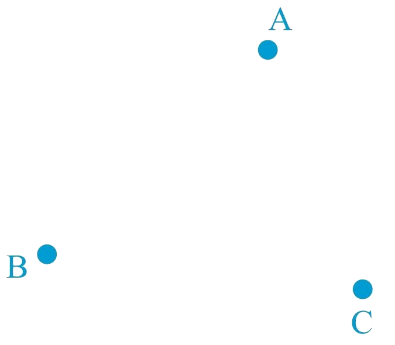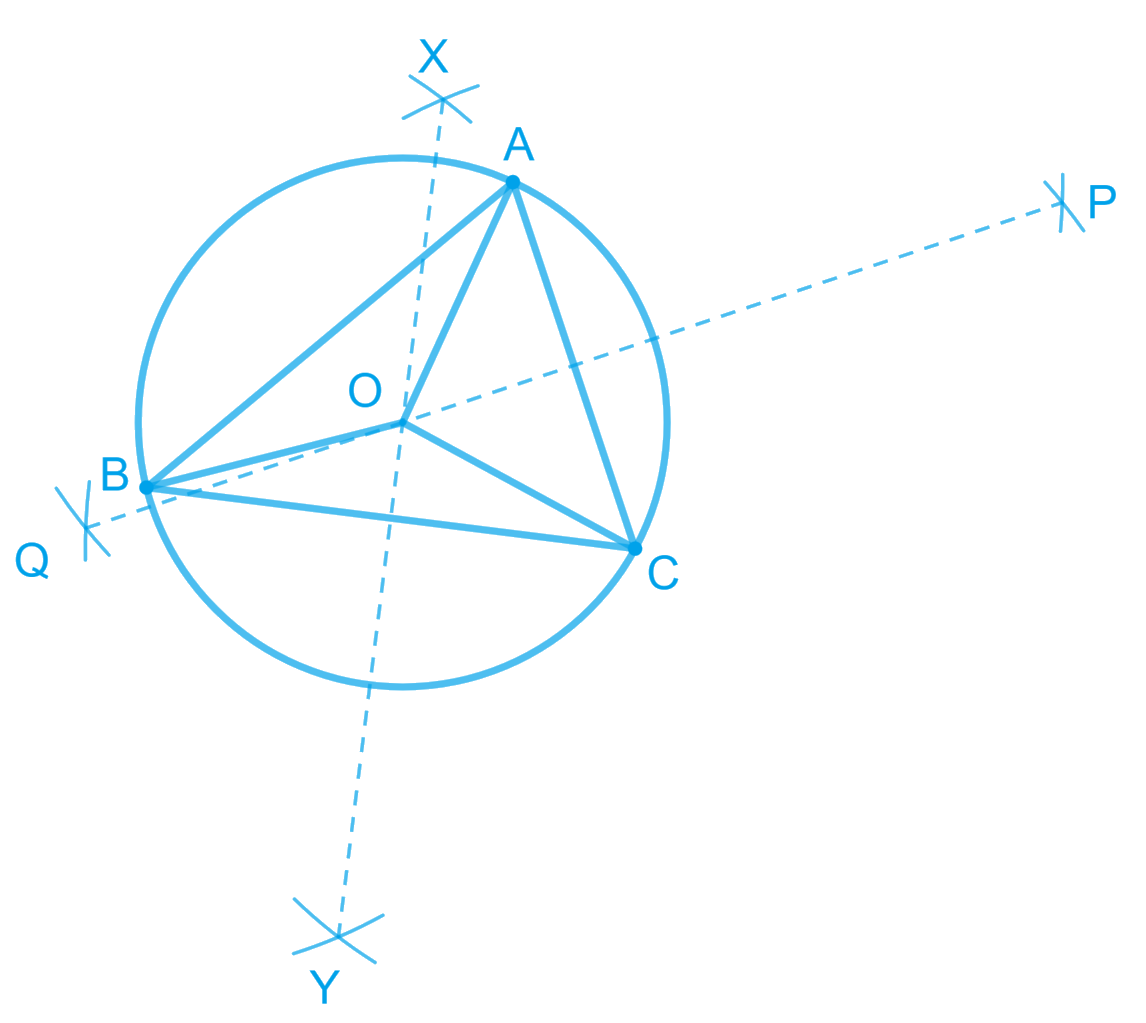# Ex.7.5 Q3 Triangles Solution - NCERT Maths Class 9

Go back to  'Ex.7.5'

## Question

In a huge park people are concentrated at three points (see the given figure)Video Solution
Triangles
Ex 7.5 | Question 3

## Text Solution

A: where there are different slides and swings for children,

B: near which a man-made lake is situated,

C: which is near to a large parking and exit.

Where should an ice-cream parlour be set up so that maximum number of persons can approach it?

(Hint: The parlor should be equidistant from $$A, B$$ and $$C$$)

Steps:

Maximum number of persons can approach the ice-cream parlour if it is equidistant from $$A, B$$ and $$C$$. Now, $$A, B$$ and $$C$$ form a triangle. In a triangle, the circumcentre is the only point that is equidistant from its vertices. So, the ice-cream parlour should be set up at the circumcentre $$O$$ of $$\Delta ABC$$.In this situation, maximum number of persons can approach it. We can find circumcentre $$O$$ of this triangle by drawing perpendicular bisectors of the sides of this triangle.

Video Solution
Triangles
Ex 7.5 | Question 3

Learn from the best math teachers and top your exams

• Live one on one classroom and doubt clearing
• Practice worksheets in and after class for conceptual clarity
• Personalized curriculum to keep up with school